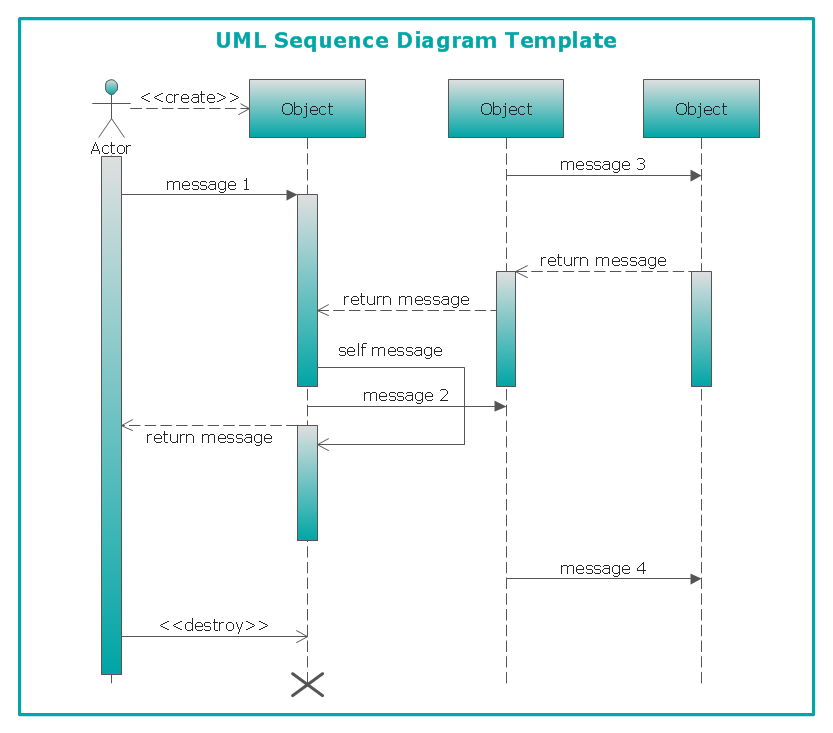This site uses cookies. By continuing to browse the ConceptDraw site you are agreeing to our Use of Site Cookies.
ConceptDraw Samples | UML Diagrams

# Rapid UML

Samples of UML 2.4 Diagrams (Activity, Class, Communication, Component, Composite Structure, Deployment, Interaction Overview, Object, Package, Sequence, State Machine, Timing, Use Case) are created using ConceptDraw DIAGRAM diagramming and vector drawing software enhanced with solution Rapid UML from ConceptDraw Solution Park. ConceptDraw DIAGRAM provides export of vector graphic multipage documents into multiple file formats: vector graphics (SVG, EMF, EPS), bitmap graphics (PNG, JPEG, GIF, BMP, TIFF), web documents (HTML, PDF), PowerPoint presentations (PPT), Adobe Flash (SWF).

## Tutorials and Solutions:

Video Tutorials: ConceptDraw Solution Park

Solutions: Rapid UML for ConceptDraw DIAGRAM

## Sample 1: UML Activity Diagram

UML activity diagram sample.

This example is created using ConceptDraw DIAGRAM
diagramming and vector drawing software enhanced with
solution Rapid UML from ConceptDraw Solution Park.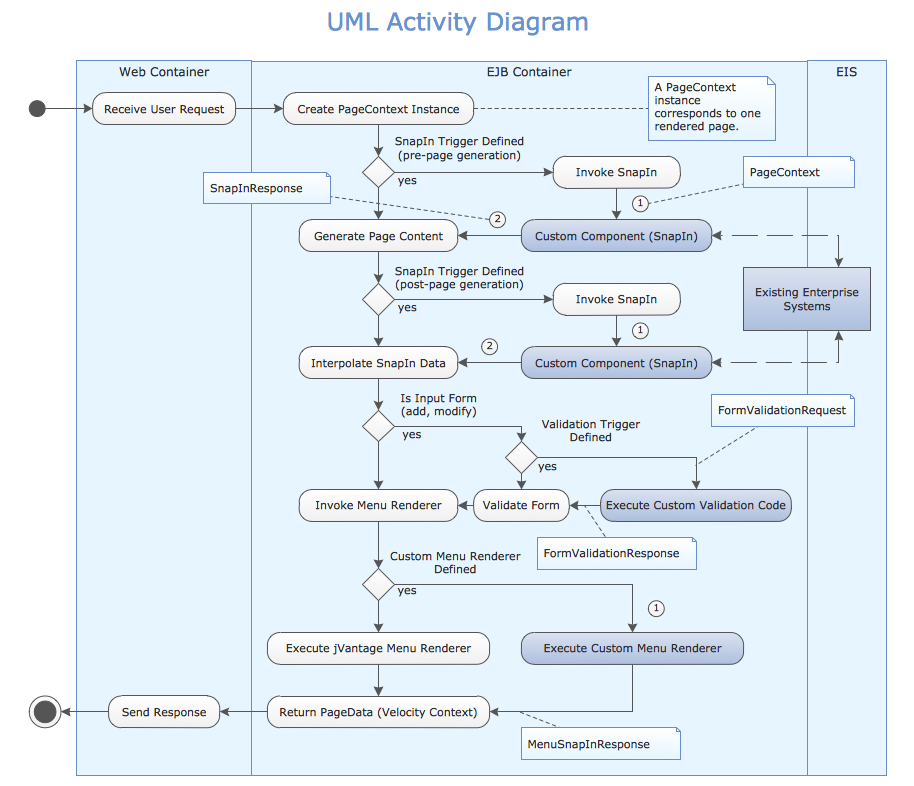## Sample 2: UML Component Diagram

UML component diagram sample.

This example is created using ConceptDraw DIAGRAM diagramming and vector drawing software enhanced with
solution Rapid UML from ConceptDraw Solution Park.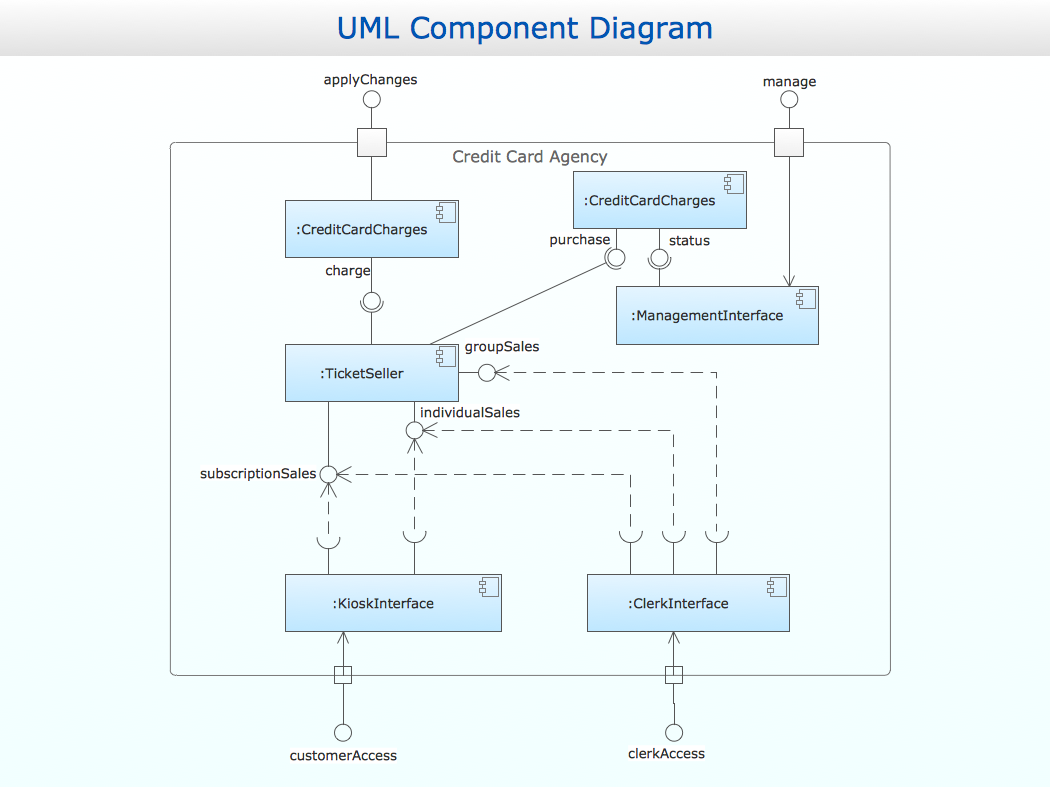## Sample 3: UML Deployment Diagram

UML deployment diagram sample.

This example is created using ConceptDraw DIAGRAM diagramming and vector drawing software enhanced with
solution Rapid UML from ConceptDraw Solution Park.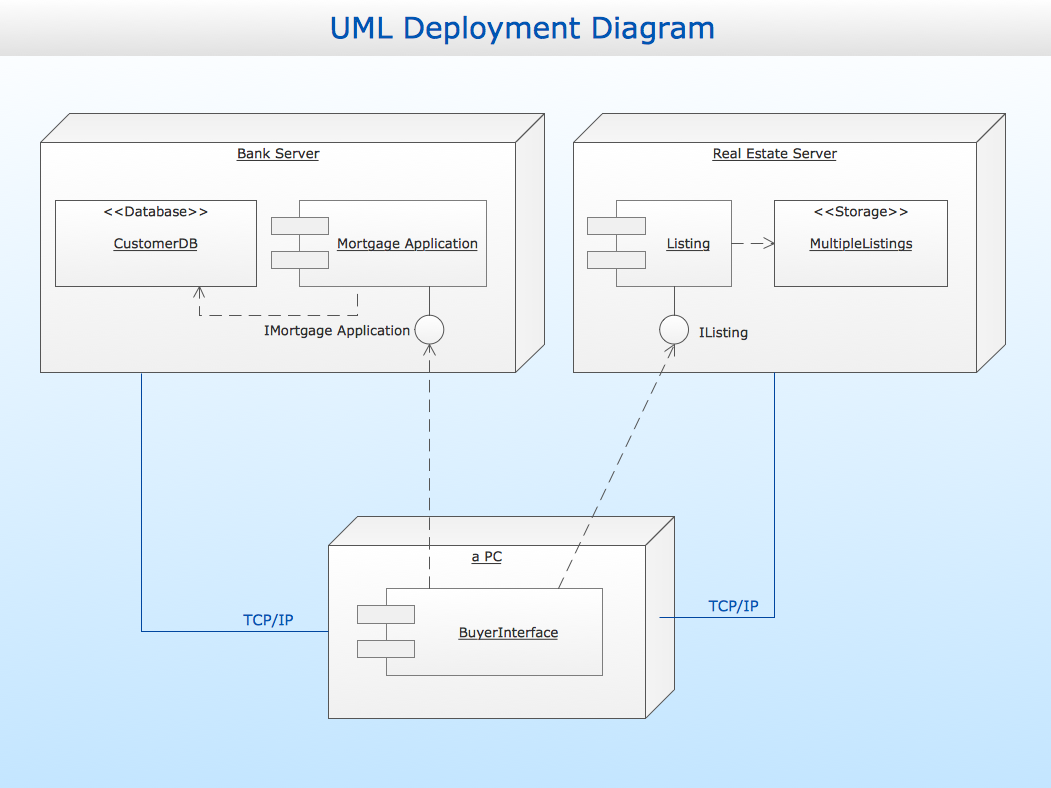## Sample 4: UML Interaction Overview Diagram

UML interaction overview diagram sample.

This example is created using ConceptDraw DIAGRAM diagramming and vector drawing software enhanced with
solution Rapid UML from ConceptDraw Solution Park.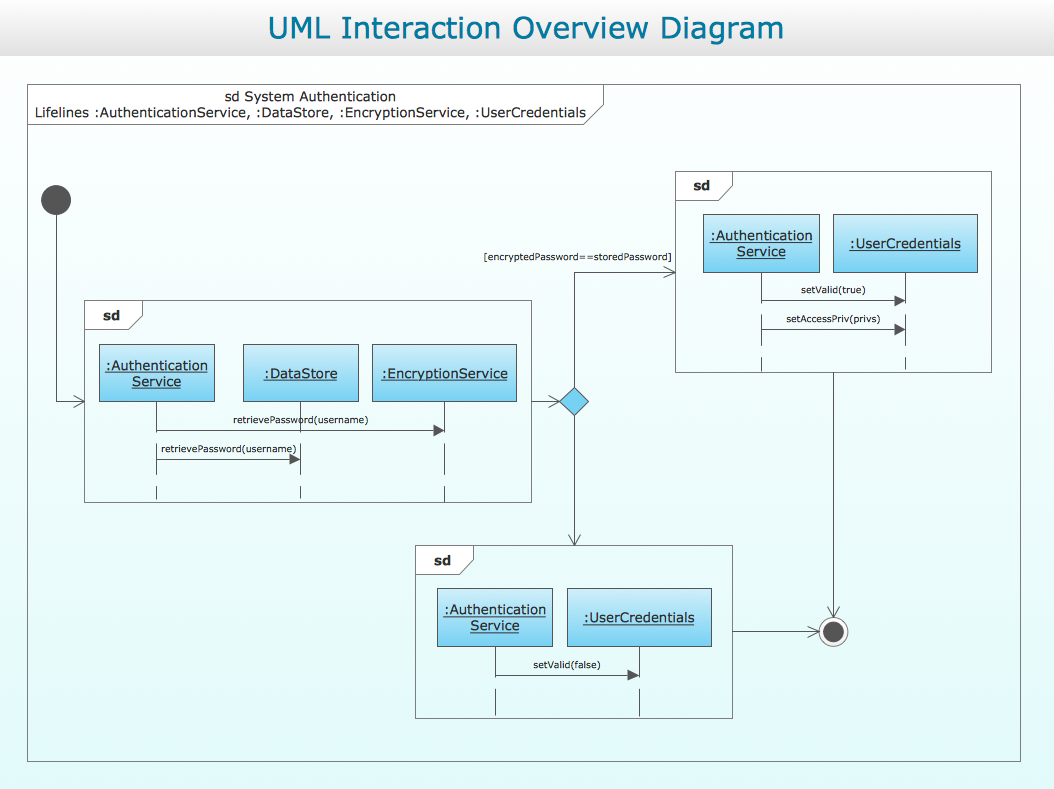## Sample 5: UML Object Diagram — Component Information Model

UML object diagram sample: Component information model.

This example is created using ConceptDraw DIAGRAM diagramming and vector drawing software enhanced with
solution Rapid UML from ConceptDraw Solution Park.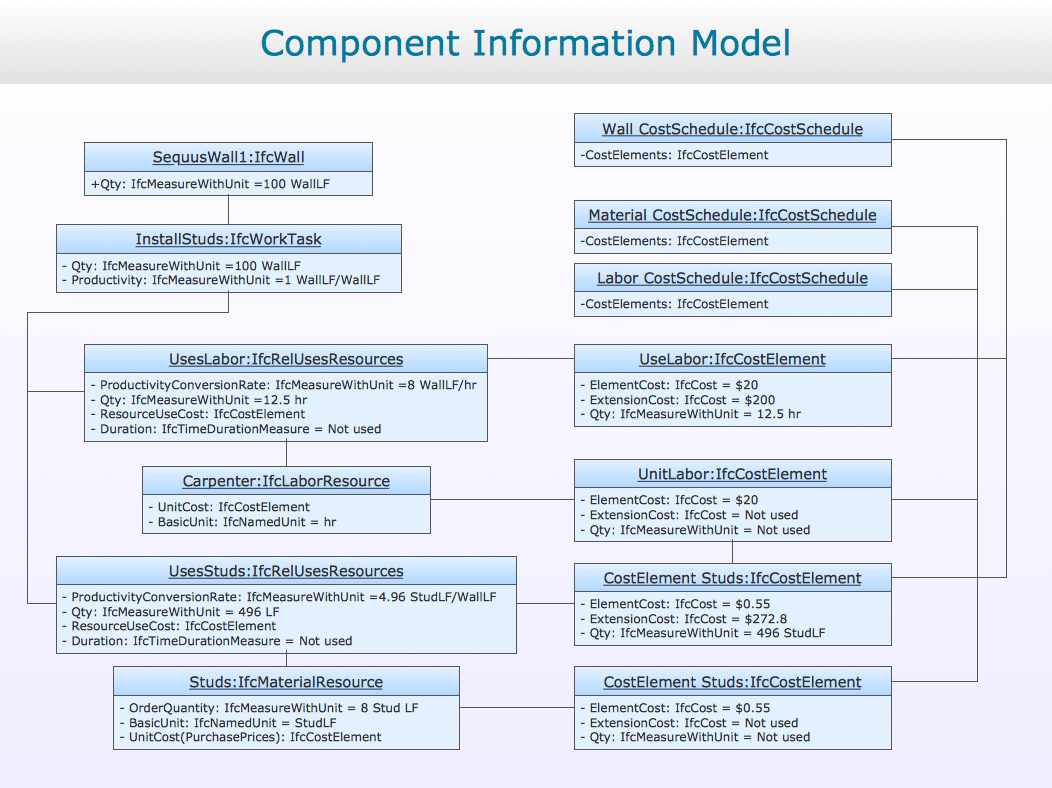## Sample 6: UML Sequence Diagram

UML sequence diagram sample.

This example is created using ConceptDraw DIAGRAM diagramming and vector drawing software enhanced with
solution Rapid UML from ConceptDraw Solution Park.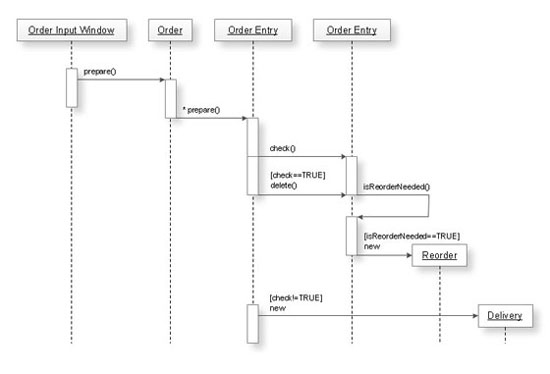## Sample 7: UML Class Diagram — Shell Objects

UML class diagram sample: Shell objects.

This example is created using ConceptDraw DIAGRAM diagramming and vector drawing software enhanced with
solution Rapid UML from ConceptDraw Solution Park.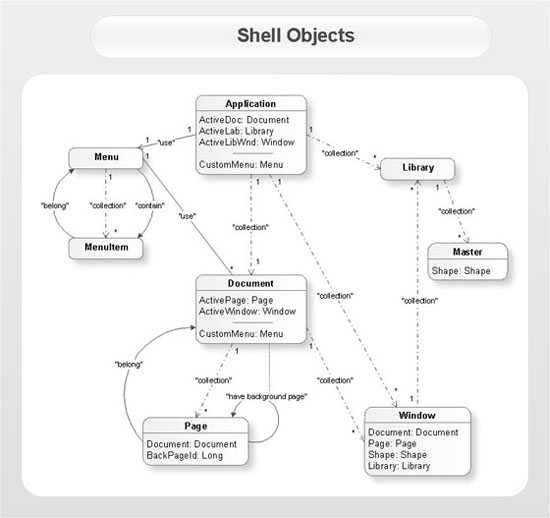## Sample 8: UML Class Diagram — Shape Object

UML class diagram sample: Shape object.

This example is created using ConceptDraw DIAGRAM diagramming and vector drawing software enhanced with
solution Rapid UML from ConceptDraw Solution Park.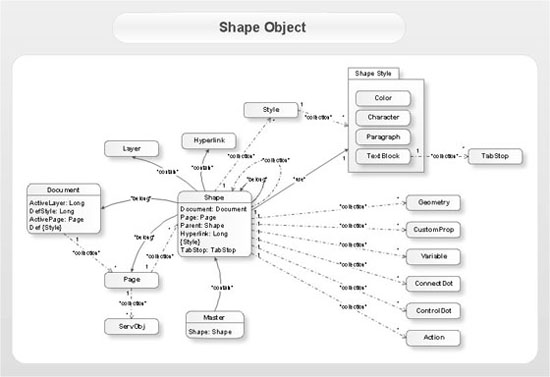## Sample 9: UML Use Case Diagram — System of Goods Selling via Catalogues

UML use case diagram sample: System of goods selling via catalogues.

This example is created using ConceptDraw DIAGRAM diagramming and vector drawing software enhanced with
solution Rapid UML from ConceptDraw Solution Park.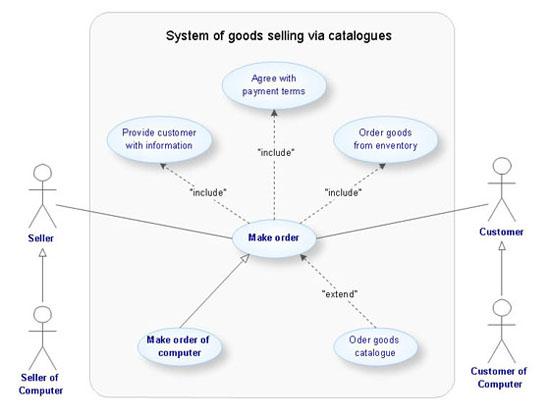## Sample 10: UML Class Diagram

UML class diagram sample.

This example is created using ConceptDraw DIAGRAM diagramming and vector drawing software enhanced with
solution Rapid UML from ConceptDraw Solution Park.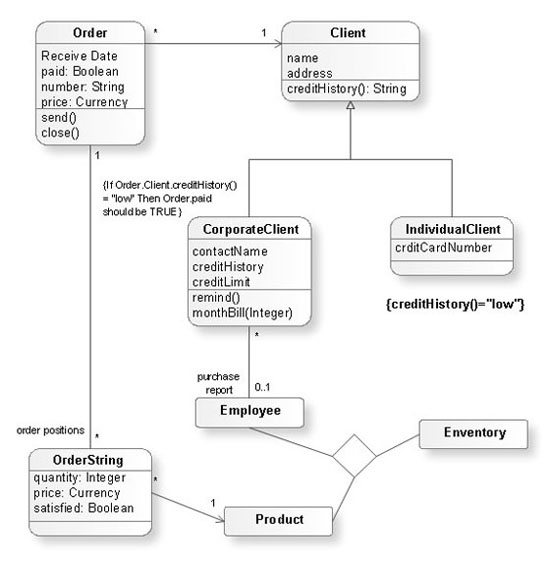## Sample 11: UML Package Diagram

UML package diagram sample.

This example is created using ConceptDraw DIAGRAM diagramming and vector drawing software enhanced with
solution Rapid UML from ConceptDraw Solution Park.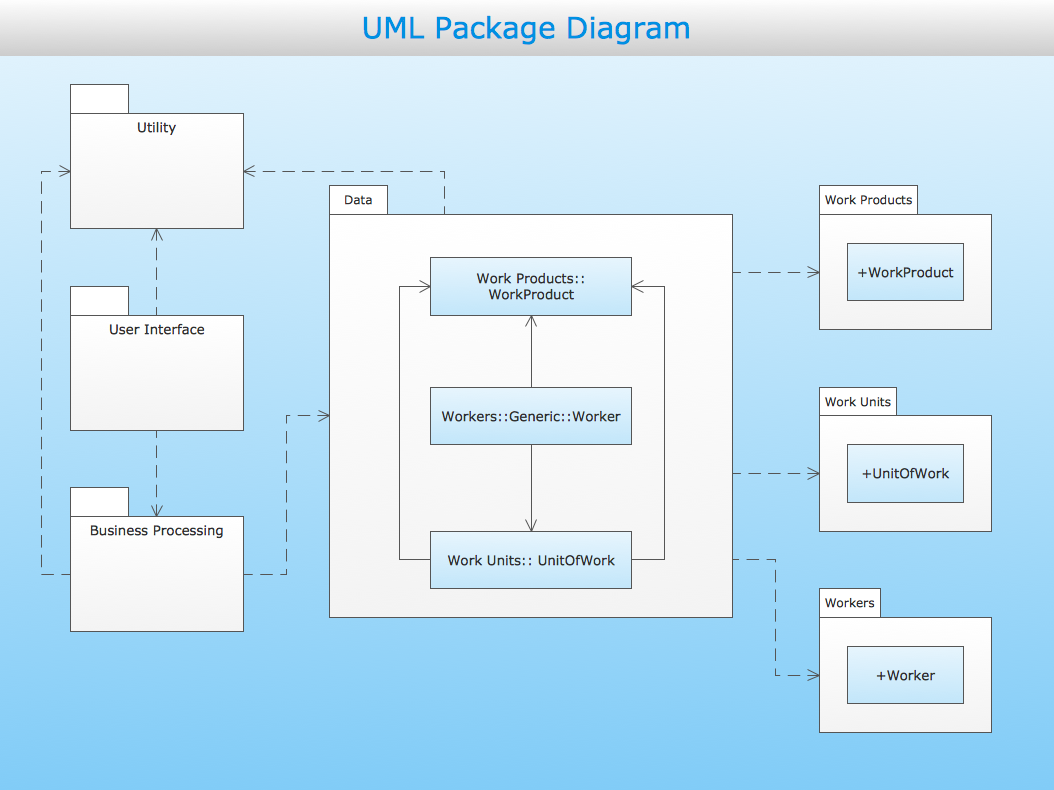## Sample 12: UML State Machine Diagram — State Transitions of RT-Component

UML state machine diagram sample: State transitions of RT-component.

This example is created using ConceptDraw DIAGRAM diagramming and vector drawing software enhanced with solution Rapid UML from ConceptDraw Solution Park.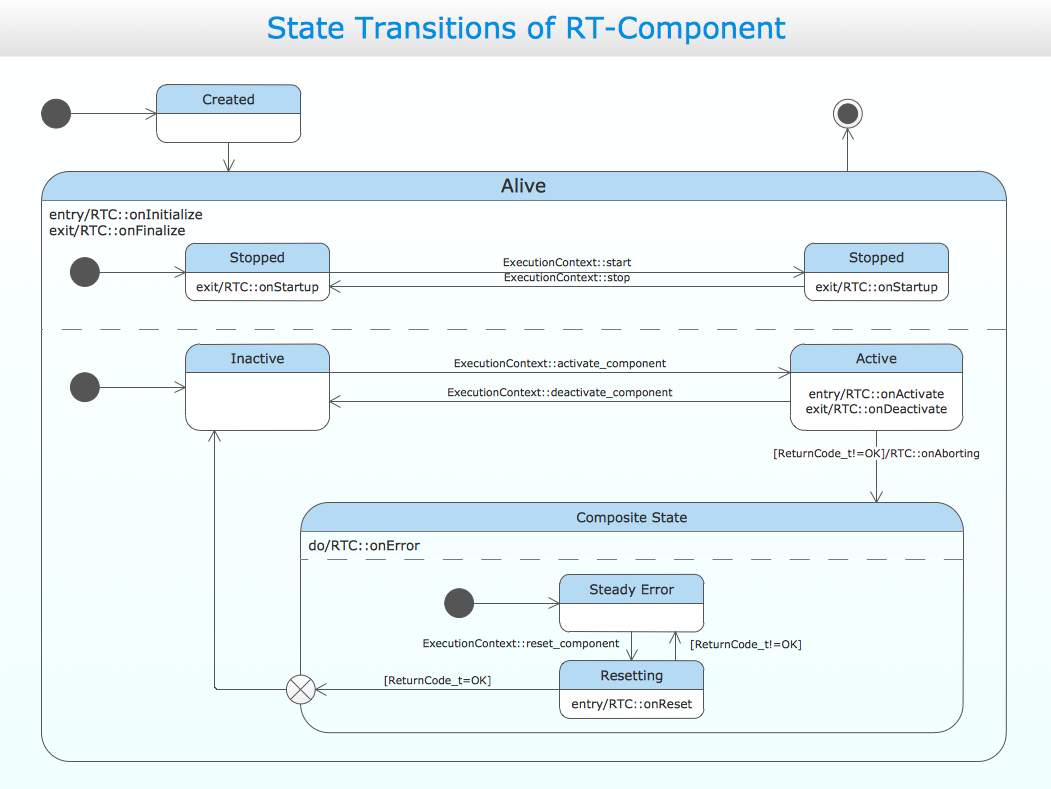## Sample 13: UML Timing Diagram

UML timing diagram sample.

This example is created using ConceptDraw DIAGRAM diagramming and vector drawing software enhanced with
solution Rapid UML from ConceptDraw Solution Park.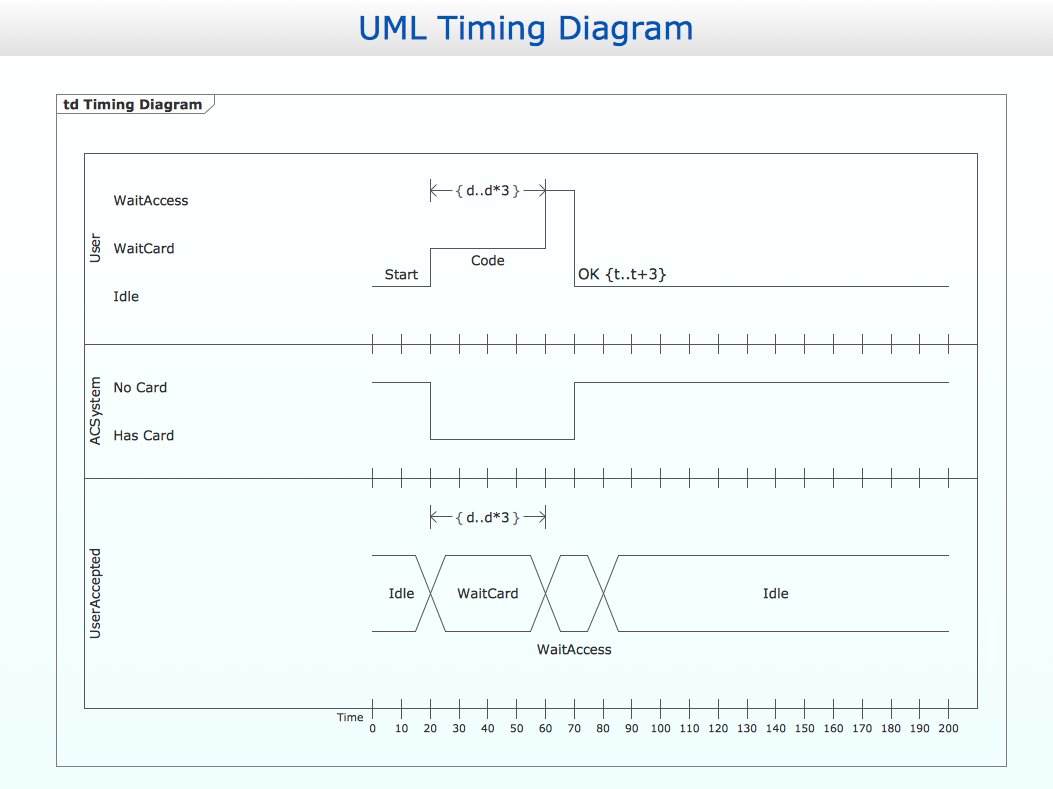## Sample 14: UML Use Case Diagram Template — System of Goods Selling via Catalogues

UML use case diagram template: System of goods selling via catalogues.

This example is created using ConceptDraw DIAGRAM
diagramming and vector drawing software enhanced with
solution Rapid UML from ConceptDraw Solution Park.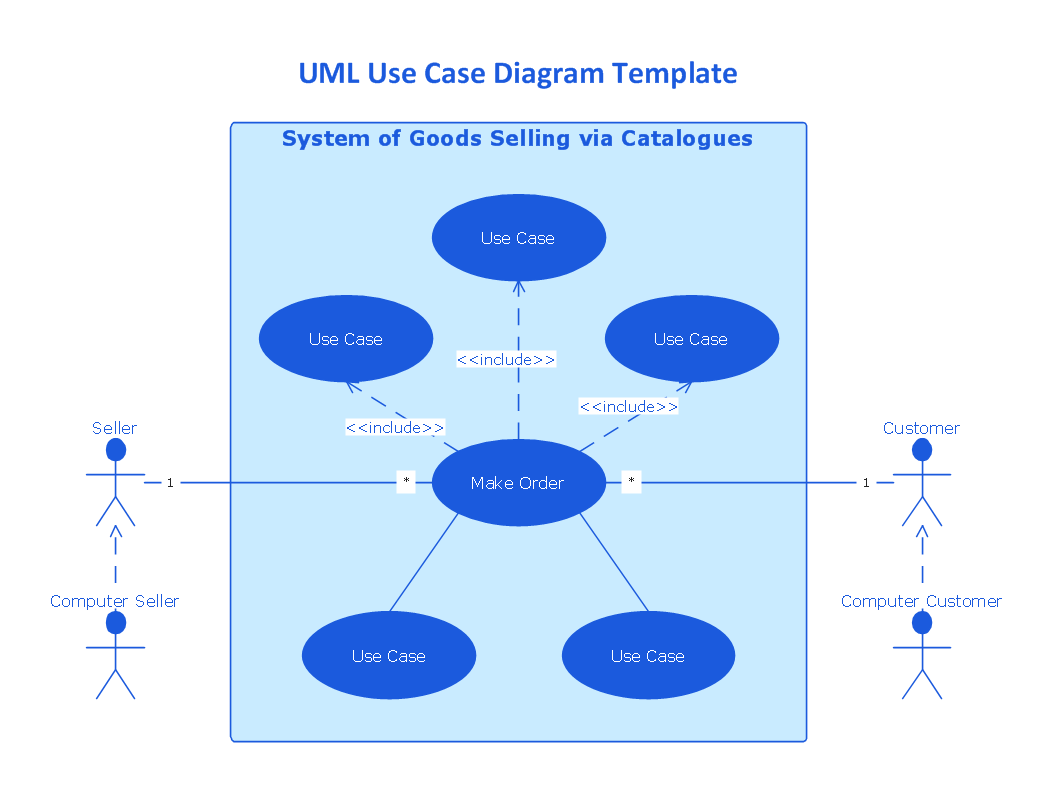## Sample 15: UML Component Diagram Template

UML component diagram template

This example is created using ConceptDraw DIAGRAM
diagramming and vector drawing software enhanced with
solution Rapid UML from ConceptDraw Solution Park.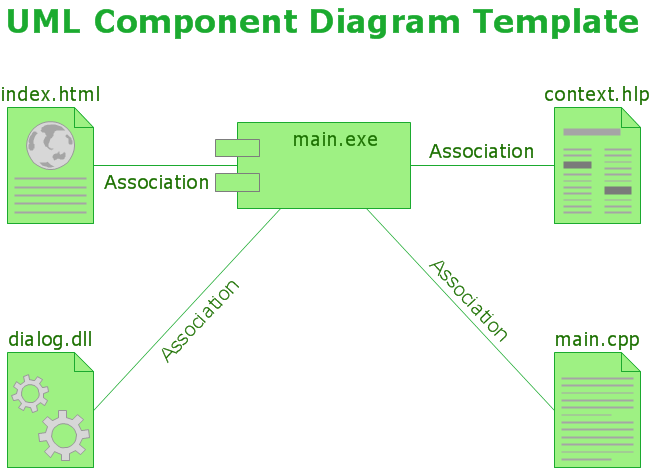## Sample 16: UML Class Diagram Template

UML class diagram template

This example is created using ConceptDraw DIAGRAM
diagramming and vector drawing software enhanced with
solution Rapid UML from ConceptDraw Solution Park.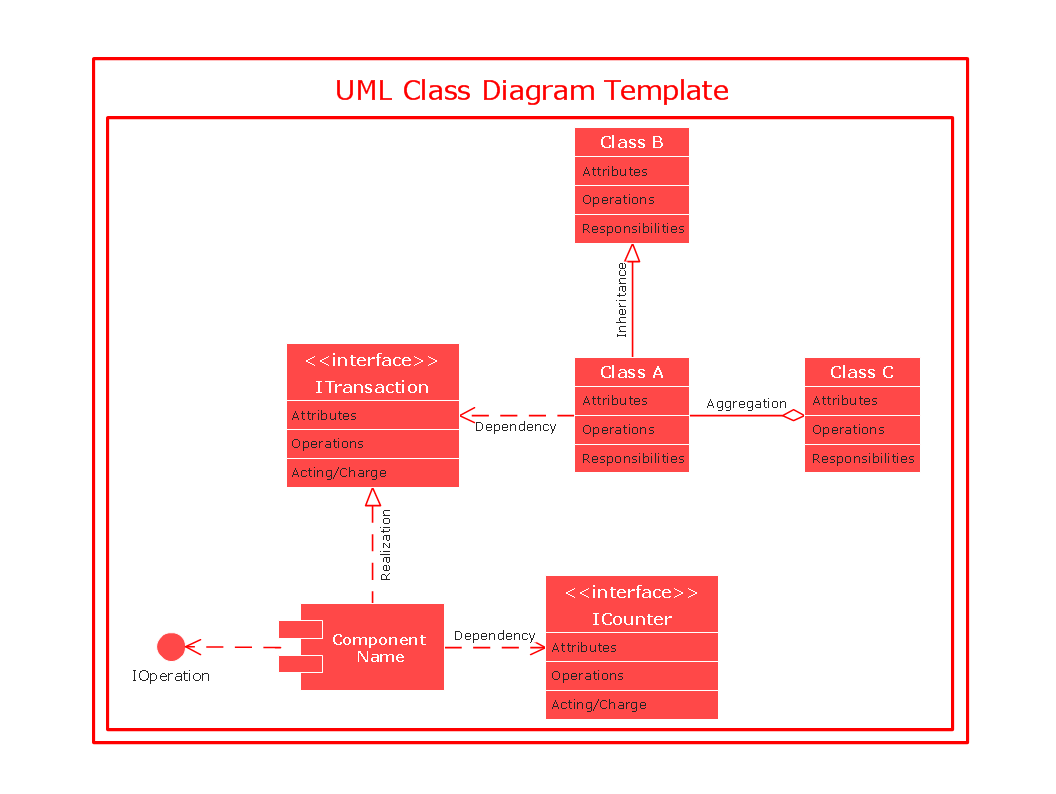## Sample 17: UML Composite Structure Diagram Template

UML composite structure diagram template

This example is created using ConceptDraw DIAGRAM
diagramming and vector drawing software enhanced with
solution Rapid UML from ConceptDraw Solution Park.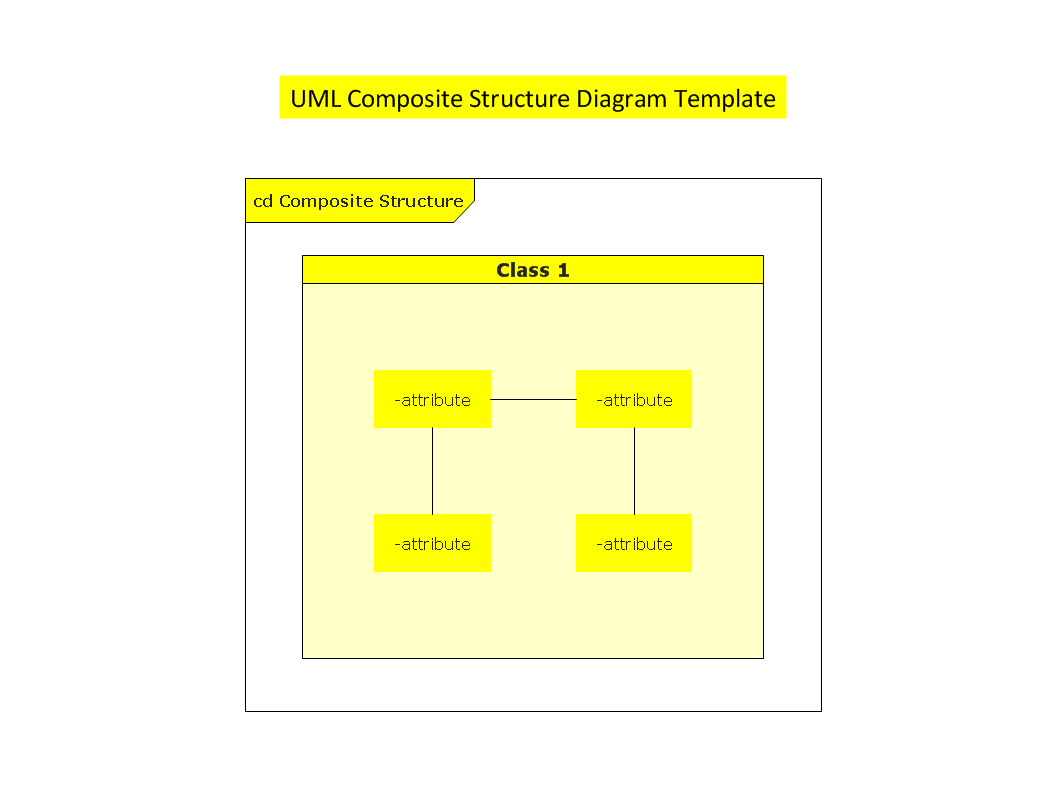## Sample 18: UML Object Diagram Template

UML object diagram template

This example is created using ConceptDraw DIAGRAM
diagramming and vector drawing software enhanced with
solution Rapid UML from ConceptDraw Solution Park.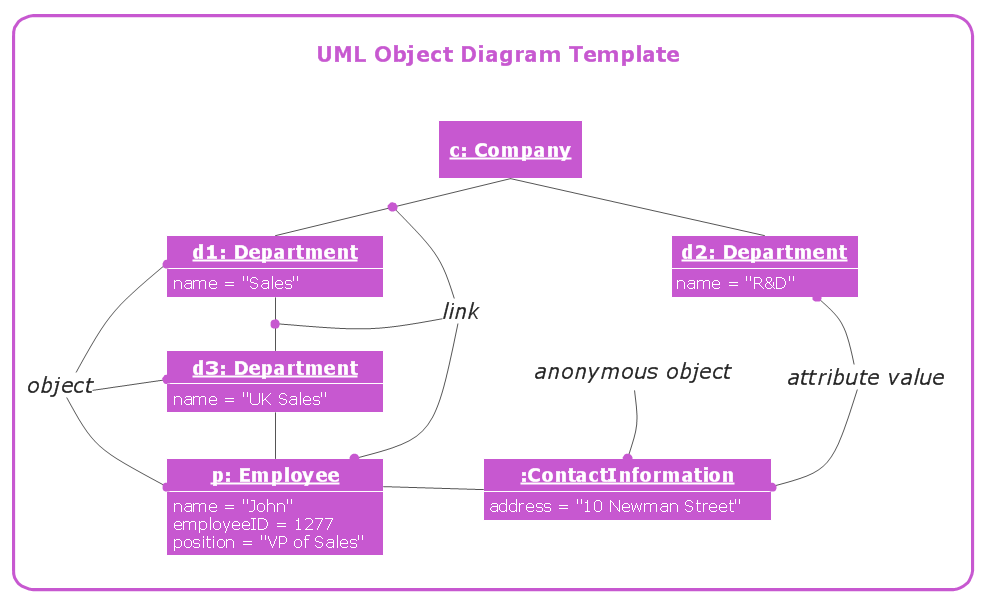## Sample 19: UML Sequence Diagram Template

UML sequence diagram template

This example is created using ConceptDraw DIAGRAM
diagramming and vector drawing software enhanced with
solution Rapid UML from ConceptDraw Solution Park.